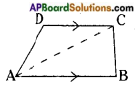# AP Board 8th Class Maths Solutions Chapter 9 Area of Plane Figures InText Questions

## AP State Syllabus 8th Class Maths Solutions 9th Lesson Area of Plane Figures InText Questions

AP State Syllabus AP Board 8th Class Maths Solutions Chapter 9 Area of Plane Figures InText Questions and Answers.

### 8th Class Maths 9th Lesson Area of Plane Figures InText Questions and Answers

Do this

Question 1.
Find the area of the following figures:     [Page No. 200]
i)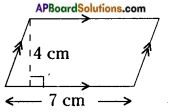Area of a parallelogram = b × h = 7 × 4 = 28 sq.cm.ii)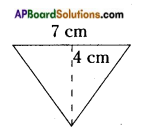Area of a triangle = $$\frac{1}{2}$$ bh = $$\frac{1}{2}$$ × 7 × 4
= 14 sq.cm.

iii)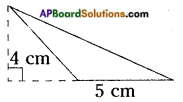Area of a triangle = $$\frac{1}{2}$$ bh = $$\frac{1}{2}$$ × 5 × 4
= 10 sq.cm.

iv)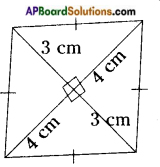Area of rhombus = $$\frac{1}{2}$$ d1d2
= $$\frac{1}{2}$$ × (4+4) × (3+3)
[∴ d1 = 4 + 4 = 8, d2 = 3 + 3 = 6]
= $$\frac{1}{2}$$ × 8 × 6
= 24 cm2

v)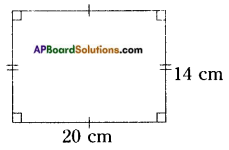Area of a rectangle = l × b
= 20 × 14 = 280 sq.cm

vi)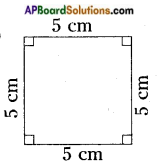Area of a square = s2
= s × s
= 5 × 5 = 25 cm2Question 2.
The measurements of some plane figures are given in the table below. However, they are incomplete. Find the missing information.     [Page No. 200]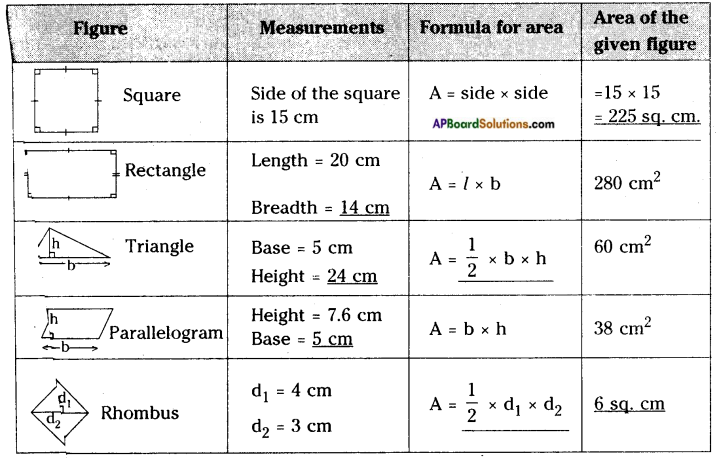Question 3.
Find the area of the following trapezium.      [Page No. 204]
fig (i)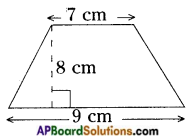Area of a trapezium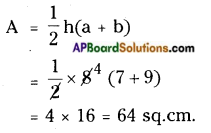fig (ii)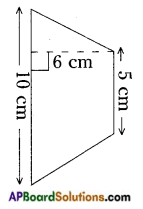Area of a trapezium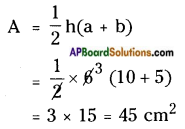Question 4.
Area of a trapezium is 16 cm2. Length of one parallel side is 5 cm and distance between two parallel sides is 4 cm. Find the length of the other parallel side. Try to draw this trapezium on a graph paper and check the area.
[Page No. 204]
Given that
Area of a trapezium = 16 sq.cm
Length of one of the parallel sides is a = 5 cm; h = 4 cm
Length of 2nd parallel side (b) = ?
A = $$\frac{1}{2}$$h(a + b)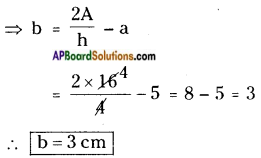Graph Sheet: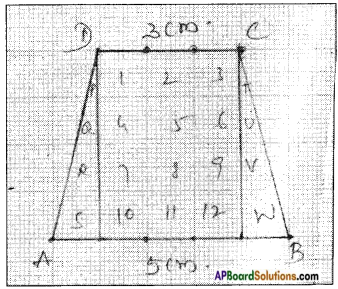Area of parallelogram ABCD = 12 sq.cm + (S + P) + (Q + R) + (W + T) + (V + U)
= 12 + 1 + 1 + 1 + 1
= 12 + 4
= 16 sq.cm

Question 5.
ABCD is a parallelogram whose area is 100 sq.cm. P is any point insile the parallelogram (see fig.) find tie area of △APB + △CPD.       [Page No. 204]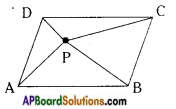Area of parallelogram ABCD = 100 sq.cm
From the given figure,
ar (△APB) + ar (△CPD) = ar (△PD) + ar (△BPC)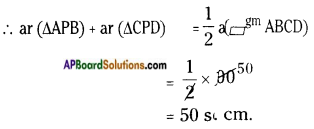Question 6.
The following details are noted in meters in the field book of a surveyor. Find the area of the fields.     [Page No. 213]
i)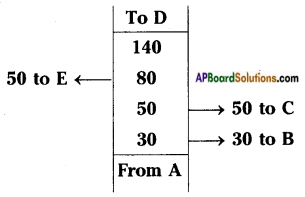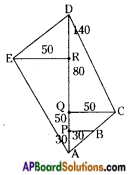From the above figure
i) A, B, C, D, E are the vertices of pentagonal field,
iii) Now the area of the field = Areas of 4 triangles and a trapezium.
PQ = AQ – AP = 50 – 30 = 20
QD = AD – AQ = 140 – 50 = 90
RD = AD – AR = 140 – 80 = 60
Area of △APB: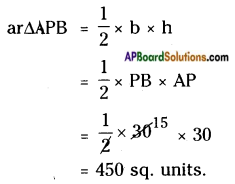Area of trapezium PBCQ: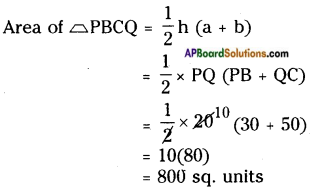Area of △QCD: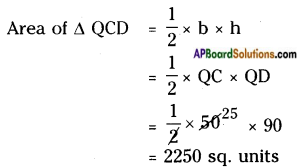Area of △DER: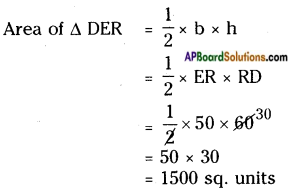Area of △ERA: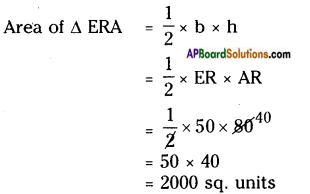∴ Area of the field = ar △APB + ar trapezium PBCQ + ar △QCD + ar △DER + ar △ERA
= 450 + 800 + 2250 + 1500 + 2000 = 7000 sq. units
ii)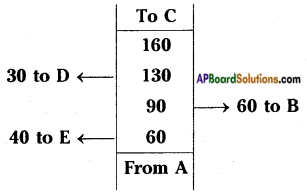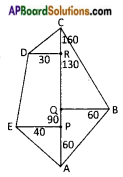From the above figure
i) A, B, C, D, E are the vertices of a pentagonal field.
ii) AC is the diagonal.
iii) The area of a field is equal to areas of 4 triangles and a trapezium.
QC = AC – AQ = 160 – 90 = 70
RC = AC – AR = 160 – 130 = 30
PR = AR – AP = 130 – 60 = 70
Area of △AQB: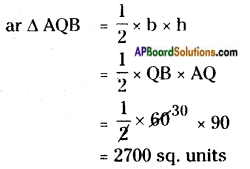Area of △QBC :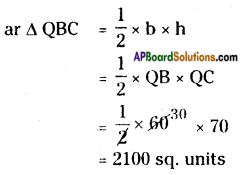Area of △DRC :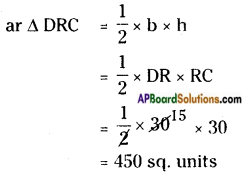Area of trapezium EPRD: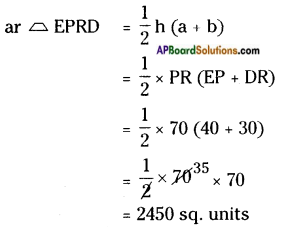Area of △EPA :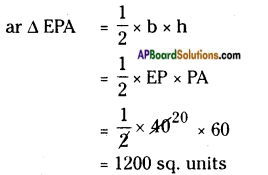∴ Area of the field = ar △AQB + ar △QBC + ar △DRC + ar trapezium EPRD + ar △EPA
= 2700 + 2100 + 450 + 2450 + 1200 = 8900 sq. unitsTry these

Question 1.
We know that parallelogram is also a quadrilateral. Let us split such a quadrilateral into two triangles. Find their areas and subsequently that of the parallelogram. Does this process in turn with the formula that you already know?   [Page No. 209]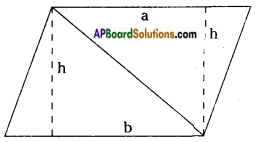Area of a parallelogram ABCD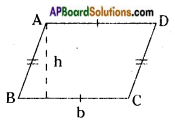Area of parallelogram ABCD
= base x height
= bh sq. units
(OR)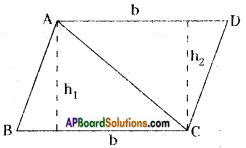Area of parallelogram ABCD
= ar △ABC + ar △ACD
= $$\frac{1}{2}$$ BC × h1 + $$\frac{1}{2}$$ AD × h2
= $$\frac{1}{2}$$ bh + $$\frac{1}{2}$$ bh [∵ h1 = h2]
= bh sq. units.
∴ This process in turn with already known formula.

Question 2.
Find the area of following quadrilaterals.      [Page No. 213]
i)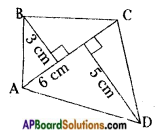d = 6 cm, h1 = 3 cm, h2 = 5 cm
= $$\frac{1}{2}$$d(h1 + h2)
= $$\frac{1}{2}$$ × 6 (3 + 5) = 3(8) = 24 cm2

ii)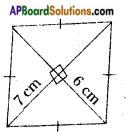d1 = 7 cm; d2 = 6 cm
Area of a rhombus A = $$\frac{1}{2}$$ d1d2
= $$\frac{1}{2}$$ × 7 × 6
= 7 × 3 = 21 cm2

iii)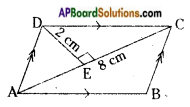Area of a parallelogram (A) = bh
(∵ The given fig. is a parallelogram in which two opposite sides are parallel)
Area of a parallelogram = 2 ar AADC
= 2 × $$\frac{1}{2}$$ × 8 × 2 = 16 Sq. cm.
[∵ Area of a parallelogram = ar △ADC + ar △ABC. But ar △ABC = ar △ADC]Question 3.
i) Divide the following polygon into parts (triangles and trapezium) to find out its area.     [Page No. 214]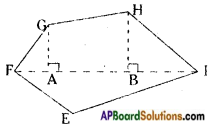FI is a diagonal of polygon EFGHI.
If perpendiculars GA, HB are drawn on the diagonal FI, then the given figure pentagon is divided into 4 parts.
∴ Area of a pentagon EFGHI = ar △AFG + ar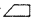AGHB + ar △BHI + ar △EFI.NQ is a diagonal of polygon MNOPQR. Here the polygon is divided into two parts.
∴ Area of a hexagon MNOPQR = ar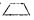NOPQ + arMNQR.

ii) Polygon ABCDE is divided into parts as shown in the figure. Find the area.     [Page No. 215].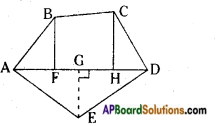If AD = 8 cm, AH = 6 cm, AF = 3 cm and perpendiculars BF = 2 cm, GH = 3 cm and EG = 2.5 cm.
Area of polygon ABCDE = ar △AFB + arFBCH + ar △HCD + ar △AED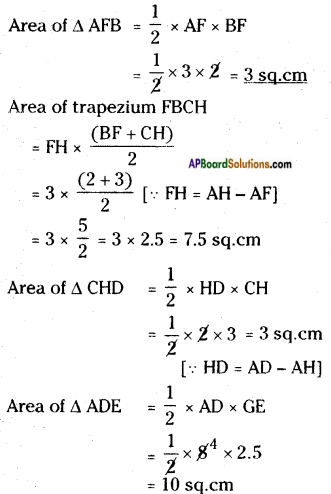So, the area of polygon ABCDE = 3 + 7.5 + 3 + 10 = 23.5 sq.cmiii) Find the area of polygon MNOPQR if MP = 9 cm, MD = 7 cm, MC = 6 cm, MB = 4 cm, MA = 2 cm.   [Page No. 215].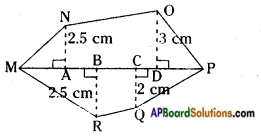NA, OD, QC and RB are perpendiculars to diagonal MP.
Area of MNOPQR
= ar △MAN + arADON + ar △DOP + ar △CQP + arBCQR + ar △MBR
Hence CP = MP – MC = 9 – 6 = 3 cm
BC = MC – MB = 6 – 4 = 2 cm
AB = MB – MA = 4 – 2 = 2 cm
DP = MP – MD = 9 – 7 = 2 cm
AD = MD – MA = 7 – 2 = 5 cm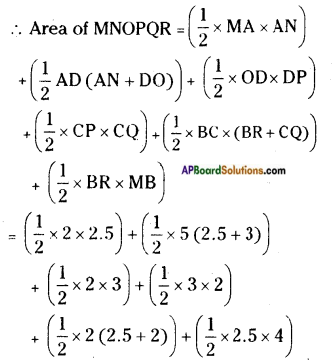= 2.5 + (2.5 × 5.5) + 3 + 3 + 4.5 + (2 × 2.5)
= 2.5 + 13.75 + 3 + 3 + 4.5 + 5
= 31.75 sq.cms

Think, discuss and write

Question 1.
A parallelogram is divided into two congruent triangles by drawing a diagonal across it. Can we divide a trapezium into two congruent triangles?    [Page No. 213]